# Hamming codes

September 2000

Hamming codes are linear binary codes. The data is split into blocks and each block has check digits assigned to it. A block of data will be of length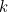, to which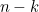check digits are assigned, so the length of data transmitted is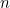digits. They use modulo 2 arithmetic. Multiplication is done as usual so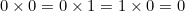and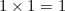. Addition is mostly as usual with 0+0=0 and 0+1=1+0=1 but there is the one exception of 1+1=0.

Let the block of data of length(ie the original message) be represented by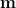. Then the codeword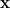will be such that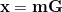where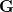is the generating matrix.

The generating matrix needs to be in the form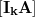to ensure that after the multiplication, the firstdigits of the codeword are the original message and they are then followed by the check digits.

Then there is the parity check matrix,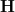, such that when multiplied by the transpose of any codeword, the transpose of the product (known as the syndrome) will be zero: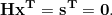From this it can be shown that when using a generating matrix in the form, the parity check matrix must have the form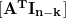.

Each pair of columns in the parity check matrix must be linearly independent. This means that there cannot be an zero column and they must all be different.

The distance between two codewords is defined as the number of places where the digits of the two words differ. For example, the distance between 0000000 and 0100010 is 2. The minimum distance between codewords in Hamming codes is 3. This means that only single errors can be detected. If two errors occurred in one codeword, then it would end up closer to a different valid codeword than the one that it had originated from and so it would be assumed it had come from the other one.

With Hamming codes,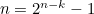, so if we were to create a code with 3 check digits appended to each message,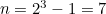. So the original messages could be of length four.

Our parity check matrix could bewhich would make G equal toLetting m be , x = mG = .

If an error occurred and x is replaced by x' = , then Hx'T =.

The fact that this is not zero shows us that an error has occurred, and that the transpose of the syndrome is the same as the fourth column of the parity check matrix tells us that the error was with the fourth digit. Therefore, by changing the fourth digit, we have the correct codeword again of . Then we just have to remove the last three digits to know what message was sent.

To see how this works, looking at G, we knew that the first four digits of the codeword were going to be the message since the identity was being used. Then, since we are using modulo 2 arithmetic, we can see that the fifth digit is the sum of the second, third and fourth digits, the sixth digit is the sum of the first, third and fourth digits and the seventh digit is the sum of the first, second and fourth digits.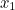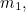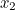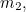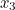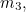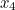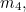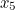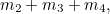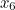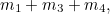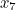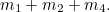Then, looking at the parity check matrix, it can be seen what sums are involved in finding the syndrome: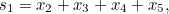but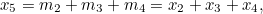so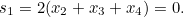Similarly,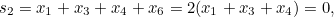and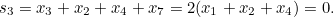So if, as in our example, an error occurs in the fourth position, it is important to remember that the fifth, sixth and seventh digits were calculated using the true value of the fourth digit. All three zeros in the syndrome rely on the fourth digit of the codeword being equal to the fourth digit that was used to calculate the extra digits so, since this is not the case, it ends up with three ones which then matches the fourth column of the parity check matrix.

back to main article

### Reference

http://web.syr.edu/~rrosenqu/ecc/main.htm